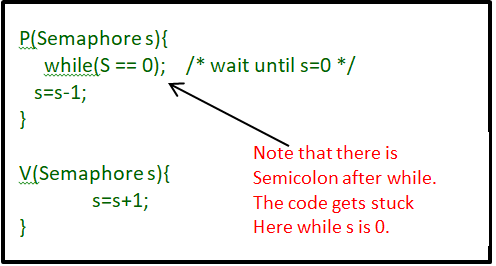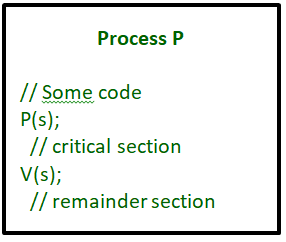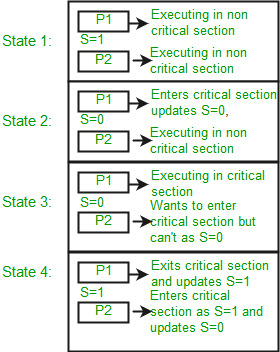# Semaphores in Process Synchronization

Prerequisite: process-synchronization, Mutex vs Semaphore

Semaphore was proposed by Dijkstra in 1965 which is a very significant technique to manage concurrent processes by using a simple integer value, which is known as a semaphore. Semaphore is simply a variable which is non-negative and shared between threads. This variable is used to solve the critical section problem and to achieve process synchronization in the multiprocessing environment.

Semaphores are of two types:

1. Binary Semaphore – This is also known as mutex lock. It can have only two values – 0 and 1. Its value is initialized to 1. It is used to implement the solution of critical section problem with multiple processes.
2. Counting Semaphore – Its value can range over an unrestricted domain. It is used to control access to a resource that has multiple instances.

Now let us see how it do so.

First, look at two operations which can be used to access and change the value of the semaphore variable.Some point regarding P and V operation

1. P operation is also called wait, sleep or down operation and V operation is also called signal, wake-up or up operation.
2. Both operations are atomic and semaphore(s) is always initialized to one.Here atomic means that variable on which read, modify and update happens at the same time/moment with no pre-emption i.e. in between read, modify and update no other operation is performed that may change the variable.
3. A critical section is surrounded by both operations to implement process synchronization.See below image.critical section of Process P is in between P and V operation.Now, let us see how it implements mutual exclusion. Let there be two processes P1 and P2 and a semaphore s is initialized as 1. Now if suppose P1 enters in its critical section then the value of semaphore s becomes 0. Now if P2 wants to enter its critical section then it will wait until s > 0, this can only happen when P1 finishes its critical section and calls V operation on semaphore s. This way mutual exclusion is achieved. Look at the below image for details which is Binary semaphore.Implementation of binary semaphores:

 `struct` `semaphore { ` `    ``enum` `value(0, 1); ` ` `  `    ``// q contains all Process Control Blocks (PCBs) ` `    ``// corresponding to processes got blocked ` `    ``// while performing down operation. ` `    ``Queue q; ` ` `  `} P(semaphore s) ` `{ ` `    ``if` `(s.value == 1) { ` `        ``s.value = 0; ` `    ``} ` `    ``else` `{ ` `        ``// add the process to the waiting queue ` `        ``q.push(P) ` `            ``sleep(); ` `    ``} ` `} ` `V(Semaphore s) ` `{ ` `    ``if` `(s.q is empty) { ` `        ``s.value = 1; ` `    ``} ` `    ``else` `{ ` ` `  `        ``// select a process from waiting queue ` `        ``q.pop(); ` `        ``wakeup(); ` `    ``} ` `} `

The description above is for binary semaphore which can take only two values 0 and 1 and ensure the mutual exclusion. There is one other type of semaphore called counting semaphore which can take values greater than one.

Now suppose there is a resource whose number of instance is 4. Now we initialize S = 4 and rest is same as for binary semaphore. Whenever process wants that resource it calls P or wait function and when it is done it calls V or signal function. If the value of S becomes zero then a process has to wait until S becomes positive. For example, Suppose there are 4 process P1, P2, P3, P4 and they all call wait operation on S(initialized with 4). If another process P5 wants the resource then it should wait until one of the four processes calls signal function and value of semaphore becomes positive.

Limitations

1. One of the biggest limitation of semaphore is priority inversion.
2. Deadlock, suppose a process is trying to wake up another process which is not in sleep state.Therefore a deadlock may block indefinitely.
3. The operating system has to keep track of all calls to wait and to signal the semaphore.

Problem in this implementation of semaphore

Whenever any process waits then it continuously checks for semaphore value (look at this line while (s==0); in P operation) and waste CPU cycle. To avoid this another implementation is provided below.
Implementation of counting semaphore

 `struct` `Semaphore { ` `    ``int` `value; ` ` `  `    ``// q contains all Process Control Blocks(PCBs) ` `    ``// corresponding to processes got blocked ` `    ``// while performing down operation. ` `    ``Queue q; ` ` `  `} P(Semaphore s) ` `{ ` `    ``s.value = s.value - 1; ` `    ``if` `(s.value < 0) { ` ` `  `        ``// add process to queue ` `        ``// here p is a process which is currently executing ` `        ``q.push(p); ` `        ``block(); ` `    ``} ` `    ``else` `        ``return``; ` `} ` ` `  `V(Semaphore s) ` `{ ` `    ``s.value = s.value + 1; ` `    ``if` `(s.value <= 0) { ` ` `  `        ``// remove process p from queue ` `        ``q.pop(); ` `        ``wakeup(p); ` `    ``} ` `    ``else` `        ``return``; ` `} `

In this implementation whenever process waits it is added to a waiting queue of processes associated with that semaphore. This is done through system call block() on that process. When a process is completed it calls signal function and one process in the queue is resumed. It uses wakeup() system call.

This article is contributed by Ashish Sharma. If you like GeeksforGeeks and would like to contribute, you can also write an article using contribute.geeksforgeeks.org or mail your article to contribute@geeksforgeeks.org. See your article appearing on the GeeksforGeeks main page and help other Geeks.My Personal Notes arrow_drop_up

Article Tags :
Practice Tags :

34

Please write to us at contribute@geeksforgeeks.org to report any issue with the above content.